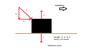# Line of motion question

• Miike012

#### Miike012

For linear motion.. is it true that a body will point in the direction of the resultant force?

This seems true to me because the I know that in circular motion, the object will fly from the point of tangency when released.
And the point of tangency for linear motion is in the direction of the motion...

Is this correct?

2nd question:
I added a picture of a lawn mower being pushed by force (P) and moving horizontally.
How would the vertical forces zero out, would this mean that the normal force is not equal to the weight force?

#### Attachments

•Q.png
6.9 KB · Views: 299

The normal force simply increases due to Py--this makes sense by Newton's Third Law. If I push down hard on a table, there's an equal and opposite force (i.e. it "pushes" up) (unless I push so hard that it breaks). So from your equation, we have N = Py + w.

I don't really know what you mean by a body "pointing". Keep in mind though, for circular motion, the acceleration points towards the center of the circle.

I meant to say.. the body's displacement is in the direction of the resultant force...

Thus is moves in the direction of Px

Is my question still not making sense?

The acceleration is always in the direction of the resultant force. When a car brakes to a stop, what is the direction of the acceleration? The resultant force? The displacement?